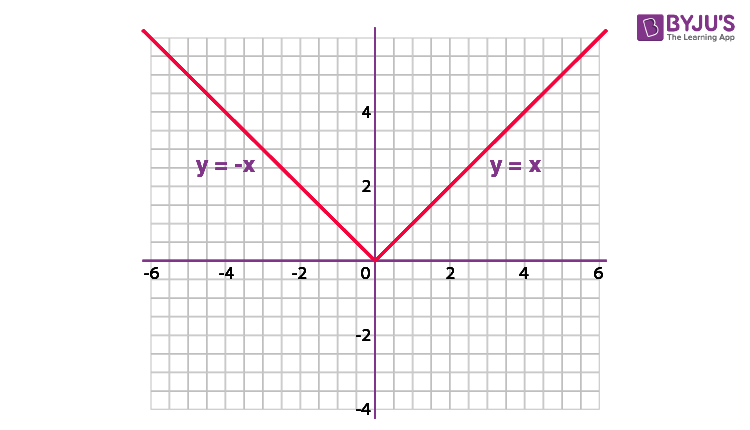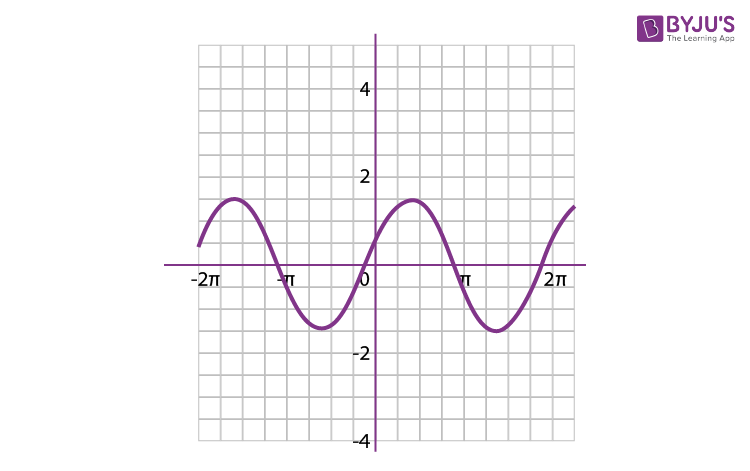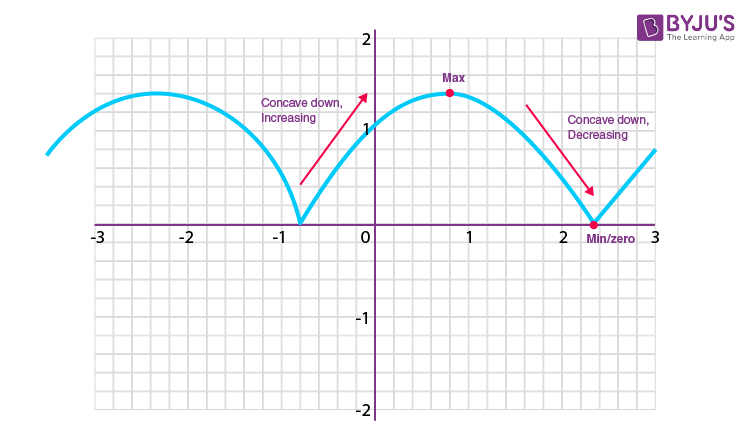Win up to 100% scholarship on Aakash BYJU'S JEE/NEET courses with ABNAT Win up to 100% scholarship on Aakash BYJU'S JEE/NEET courses with ABNAT

# Examples on Modulus Function

What Is the Modulus Function?

The modulus of a given number describes the magnitude of the number. Modulus Function is defined as the real-valued function, say f: R -> R, where y = |x| for each x ∈ R OR f(x) = |x|. This function can be defined using the modulus operation as follows:

$$\begin{array}{l}f(x) =\left\{\begin{matrix} -x &x <0 \\ x & x \geq 0 \end{matrix}\right.\end{array}$$

For each non-negative value of x, f(x) is equal to the positive value of x. But for negative values of x, f(x) is equal to the negative value of x. Read more.

## Properties of Modulus Function

The modulus function has the following properties:

1. For any real number x, we have

$$\begin{array}{l}\sqrt{x^2} = |x|\end{array}$$

2. ||x||=|x|

3. If a and b are positive real numbers, then

$$\begin{array}{l}a]\ x^2 \leq a^2 \Leftrightarrow |x| \leq a \Leftrightarrow -a \leq x \leq a\\ b] \ x^2 \geq a^2 \Leftrightarrow |x| \geq a \Leftrightarrow x \leq -a \\ c] \ x^2 > a^2 \Leftrightarrow |x| > a \Leftrightarrow x < -a \\ d]\ a^2 \leq x^2 \leq b^2 \Leftrightarrow a \leq |x| \leq b \Leftrightarrow x \epsilon [−b, −a] \cup [a, b].\\\end{array}$$

4.  If a is negative, then
a. |x| a, x in R

b. |x| ≤ a, x = ϕ

5.  For real numbers x and y

$$\begin{array}{l}a. |xy| = |x| |y|\\ b. |xy| = |x| |y|, y \neq 0|\\\end{array}$$
$$\begin{array}{l}c. |x+y| \leq |x| + |y| \text \ and \ |x−y| \leq |x| – |y| \text \ for \ x, \ y \geq 0 \text \ or \ x, \ y < 0, |x+y| = |x| + |y|\\\end{array}$$
$$\begin{array}{l}d. |x+y| \geq |x| − |y| |x+y| \text \ and |x−y| \geq |x| − |y| \text \ for \ x, \ y \geq 0 \text \ and \ |x| \geq |y| y \text \ or \ x, \ y < 0 \text \ and \ |x| \geq |y|, |x−y| = |x| − |y|\\\end{array}$$
.

6. The absolute value has the following four fundamental properties (a and b are real numbers):

$$\begin{array}{l}1] \ |a|\geq 0} \text \ Non-negativity\\ 2] \ |a|=0\iff a=0} \text \ Positive-definiteness\\ 3] \ |ab|=|a|\,|b|} \text \ Multiplicativity\\ 4] \ |a+b|\leq |a|+|b|} \text \ Subadditivity, \text specifically \ the \ triangle \ inequality\\\end{array}$$

7. Some additional useful properties are given below. These are either immediate consequences of the definition or implied by the four fundamental properties above.

a] ||a||=|a|: Idempotence (The absolute value of the absolute value is the absolute value)

$$\begin{array}{l} b] \ |-a|=|a|} \text \ Evenness \ (reflection \ symmetry \ of \ the \ graph)\\ c] \ |a-b|=0\iff a=b} \text \ Identity \ of \ indiscernibles \ (equivalent \ to \ positive-definiteness) \\ d] \ |a-b|\leq |a-c|+|c-b|} \text \ Triangle \ inequality \ (equivalent \ to \ subadditivity) \\ e] \ \left|{\frac {a}{b}}\right|={\frac {|a|}{|b|}}\ }\ (\text\ if \ b\neq 0}) \text \ Preservation \ of \ division \ (equivalent \ to \ multiplicativity) \\ f] \ |a-b|\geq {\big |}\,|a|-|b|\,{\big |}} \text\ Reverse \ triangle \ inequality \ (equivalent \ to \ subadditivity)\\\end{array}$$

8. Two other useful properties concerning inequalities are as follows:

$$\begin{array}{l}1] \ |a|\leq b\iff -b\leq a\leq b}\\ 2] \ |a|\geq b\iff a\leq -b\ } \text \ or \ a\geq b}\\\end{array}$$

## Graph of Modulus Function## Video Lesson## Solved Examples on Modulus

Example 1: Solve modulus and find the interval of x for |x2 – 5x + 6|

Solution: |x2 – 5x + 6| = |(x – 2)(x – 3)| = |f(x)|

As per the modulus definition,

|f(x)| = f(x); if f(x) is positive

| f(x) |= -f(x); if f(x) is negative

f(x) = (x – 2)(x – 3) is positive or zero when x = (- ∞, 2] ∪ [3, ∞)

f(x) = (x – 2)(x – 3) is negative when x = (2, 3)

So, |x2 – 5x + 6| = (x2 – 5x + 6) when x = (-∞, 2] ∪ [3, ∞) and

|x2 – 5x + 6| = -(x2 – 5x + 6) when x = (2, 3)

Example 2: If |x2 – 5x + 6| + |x2 – 8x + 12| = 0. Find x.

Solution:

Every modulus is a non-negative number, and if two non-negative numbers add up to get zero, then individual numbers itself equal to zero simultaneously.

x2 – 5x + 6 = 0 for x = 2 or 3

x2 – 8x + 12 = 0 for x = 2 or 6

Both the equations are zero at x = 2

So, x = 2 is the only solution for this equation.

Example 3: Solve for x, |x – 1| – |x – 2| = 10

Solution: Here, the critical points are 1 and 2.

Let us check for the values less than 1, between 1 and 2, and greater than 2.

Case 1: For x ≤ 1

-(x – 1) – {-(x – 2)} = 10

or -x + 1 + x – 2 = 10

or -1 = 10 (which is not possible)

Case 2: x ∈ (1, 2)

(x – 1) – {-(x – 2)} = 10

or x – 1 + x – 2 = 10

or 2x – 3 = 10

or x = 13/2

In this case, x ∈ (1, 2), so 6.5 is not a solution.

Case 3: x ≻ 2

(x – 1) – (x – 2) = 10

=> 1 = 10 (which is not possible)

So, this equation has no solution.

Example 4: Draw the graph of |sin(x) + cos(x)| in the interval x ϵ [0, π].

Solution:

|sin(x) + cos(x)| = |√2 sin(x + π/4)|

First, make the graph of the function, then take the modulus.

Here, at the place of sinx, we have √2 sin(x + π/4), so the graph will be of type sin x only, but the graph will be starting from x = -π/4.

y = sin(x) + cos(x)Take all negative y values to the positive y side, and positive y remains the same.

y = |sin(x) + cos(x)| = |√2 sin(x + π/4)|Example 5: Find the domain and range of the below function.
a. y = |1−x|
b. y = 2 − |1 − x|
c. y = 2√x − |x|

Solution:

a. y = |1 − x|
Clearly, this is defined for x ∈ R. So, Domain is R.
Now, |1 − x| ≥ 0 for all x ∈ R
So, the range is [0, ∞).

b. y = 2 − |1 − x|
Clearly, this is defined for x ∈ R.

So, the domain is R.
Now, |1 − x| ≥ 0 for all x ∈ R
or
−|1 − x| ≤ 0
2 − |1 − x| ≤ 2 for all x∈R
So, the range is (−∞, 2].

c. y = 2√x − |x|
Now, x− |x| = {x − x = 0 if x ≥ o, x + x = 2x if x ≤ 0

Therefore, 2√x − |x| is undefined for all x ∈ R.
So, the domain of the function is ϕ.

Q1

### What do you mean by a modulus function?

A modulus function is a function which gives the absolute value of a number or variable. The outcome of the modulus function is always positive.

Q2

### How do you find the modulus of a negative number?

The modulus of a negative number is the number without the minus sign. For example, |-4| = 4.

Q3

### Is the modulus of a number always positive?

Yes, the modulus of any number is always positive.

Q4

### What is the range of the modulus function?

The range of the modulus function is the set of non-negative real numbers, i.e., (0,∞).

Q5

### What is the shape of the graph of modulus function f(x) = |x|?

The graph of modulus function f(x) = |x| is a V-shaped graph.

Test your Knowledge on examples on modulus function